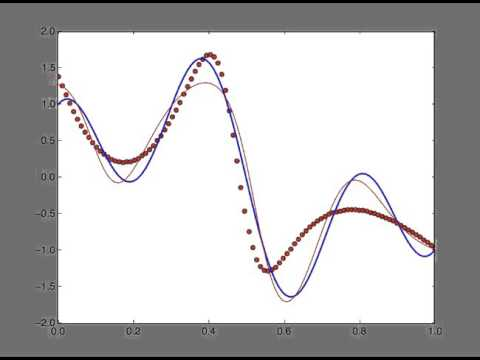# Optimization for neural network

The other day I read this article. This post was written about the variation of gradient descent algorithm. Gradient descent is an de facto standard algorithm for optimizing deep learning. Iterative optimization algorithm such as gradient descent plays an important role in deep learning because there is no way to find the global solution analytically.

A lot of research has been done to find efficient type of gradient descent for training large scale deep neural network. I heard about some of them, but I didn’t understand the detail of each algorithm. So I decided to implement small samples to run these gradient descent algorithms. This project was uploaded on GitHub. I implemented some of the algorithms actively researched and I could figure out why recent developed algorithms optimize faster and reach global minimum. I wrote the program in Python and numpy. The problem to learn was mimicking sine wave with neural network.So the program is very simple to read. I could grasps the structure of optimization algorithm and each gradient descent algorithm. I hope the program can also help you understand the variation of gradient descent algorithms recently developed.

Thank you.